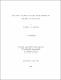## The Corona Theorem for the multiplier algebras on weighted Dirichlet spaces2009
##### Publisher
University of Alabama Libraries
##### Abstract

In this dissertation we give a proof of "The Corona Theorem for Infinitely Many Functions for the Multiplier Algebras on Weighted Dirichlet Spaces", and we obtain explicit estimates on the size of the solution. We denote the open unit disc of the complex plane by D, and for α in (0, 1) we denote by Dα the Weighted Dirichlet Spaces of all holomorphic functions on D, and by

f

α the weighted Dirichlet norm. To prove the Dα Corona Theorem and find a bound for the solution we use a similar technique employed by Trent [Tr 2] in his proof of the corona theorem for Dirichlet space. The first challenge is to find a norm that is expressed on the boundary of D. In this dissertation we first establish such a norm. The new norm basically enables us to extend multipliers on the weighted Dirichlet spaces to multipliers on a related weighted harmonic Dirichlet spaces. Finally using Schur's Theorem and Cauchy's Transform we are able to prove the the weighted Dirichlet corona theorem and give a bound for the solution.

##### Description
Electronic Thesis or Dissertation
Mathematics